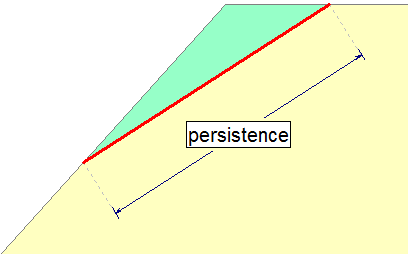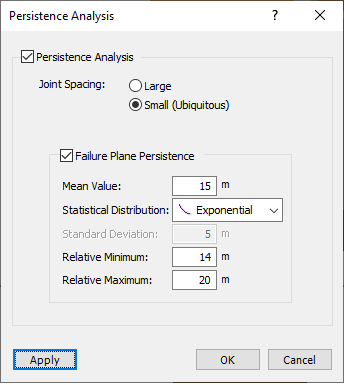# Joint Persistence Analysis

Joint Persistence Analysis is a feature of the RocPlane Probabilistic analysis that allows you to define a Statistical Distribution for joint persistence. For a given wedge size, randomly generated values of persistence can be used as a filter to determine if a wedge can actually form or to scale the wedge size down according to the allowable persistence.

The Persistence Analysis option is a refinement to the RocPlane Probabilistic analysis. It can be used on its own or simultaneously with any other Probabilistic analysis options (i.e., you can define any other input random variables, in addition to the joint persistence). In general, checking for allowable joint persistence in a probabilistic analysis can only DECREASE the calculated Probability of Failure. The Persistence Analysis. option should give more realistic estimates of Probability of Failure for slopes where the joint planes are not continuous.

## Definition of Joint Persistence for RocPlane Analysis

Since a RocPlane analysis is two-dimensional, the definition of failure plane persistence must also be two-dimensional. In the case of a planar wedge, the length of the failure plane as measured in the two-dimensional view defines the persistence. For a given wedge and a given sampled value of persistence, the length of the failure plane must be less than or equal to the persistence or else a wedge cannot form.Persistence is defined by the two-dimensional trace of the wedge failure plane

## Using the Joint Persistence Analysis Option

To use the Joint Persistence Analysis option:

1. Select Persistence Analysis from the Analysis menu.
2. In the Persistence Analysis dialog, select the Persistence Analysis check box.
3. Enter the Joint Spacing and Failure Plane Persistence information as described below.## Joint Spacing

Joint Spacing can be defined as either Large or Small:

### LARGE JOINT SPACING

With the Large Joint Spacing option, it is assumed that there is only one trace of the Failure Plane on the slope face. The daylighting point of the failure plane may occur randomly anywhere between the toe and crest of the slope, resulting in a uniform distribution of wedge height (measured vertically from the daylight point to the slope crest).

If the failure plane length exceeds the allowable persistence, the wedge cannot exist. If the failure plane length is smaller than the allowable persistence, the wedge can form and a Factor of Safety is calculated.

The Large Joint Spacing option is a lower bound solution for Probability of Failure because the spacing and persistence condition limit the formation of wedges.

### SMALL JOINT SPACING (UBIQUITOUS JOINTS)

The Small Joint Spacing option assumes ubiquitous joints (i.e., joints are numerous and may occur at any location). This model automatically scales down the wedge size until the persistence conditions are met. So a wedge is almost always formed in each simulation if the geometry of the failure plane and slope creates a kinematically feasible wedge. Its size is dependent on the sampled persistence and the geometry of the bench.

The Small Joint Spacing option is an upper bound solution for probability of failure, because a wedge will always be created independent of any spatial location of the failure plane on the slope face. The only thing that limits the size of the wedge is the geometry of the bench and the persistence of the joint.

## Failure Plane Persistence

To define the Failure Plane Persistence, select the check box, enter a Mean Value of persistence, select a Statistical Distribution, and enter the Standard Deviation, Relative Minimum, and Relative Maximum values.

NOTE: If the Failure Plane Persistence check box is NOT selected, joint persistence is assumed to be infinite.

For further information and practical examples, see Tutorial 06 - Bench Design in RocPlane and Persistence and Bench Design in the RocPlane Theory Documents.

## Bench Design

Persistence Analysis is an integral part of the Bench Design option in RocPlane. When using Persistence Analysis with Bench Design, the persistence data is input through the Bench Design dialog rather than the Analysis menu.

NOTE: The Persistence Analysis option cannot be used in conjunction with the Scale Wedge option. If the Persistence Analysis option is in use, the Scale Wedge option is NOT available, and vice-versa.